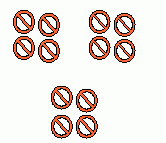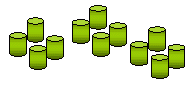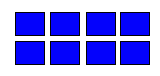## D Is 4 Division (Math)

D is 4 D-I-V-I-S-I-O-N,

Which makes math a lovely function.

Division is fun,

If u r its fan.

Any number is divided by 1,

The answer is the same one.

An even number is divided by 2,

U get half of the number, true!

An odd number is divided by 2,

U end up with a reminder, not cool,

Any number is divided by itself,

The answer is 1, what fun!

Division is the inverse of  multiplication,

Practice makes perfection.

If C is divided by A equal to B,

Then A times B is equal to C,

And B times A is also equal to C.

For instance,

100 is divided by 4 is 25,

4 times 25 is 100,

25 times 4 is also 100.

Division is tough,

If u don’t work hard enough…

I love divisions,

Because it is cool,

Work on the below versions,

Divisions love U!

*****

The poem is originally written by Jingle as an entry 4

ABC Wednesday, D Is 4 Division!!

The following practices come from Reference: http://www.kwiznet.com/

Practices 2 learn Divisions Method:Q 1: In the picture below 6 stars are divided into 3 groups with ___ in each group.3 2 1 Q 2: In the picture below 8 medals are divided into 4 groups with ___ in each group.2 4 3 Q 3: In the picture below 12 cylinders are divided into ___ groups with 4 cylinders in each group.6 4 3 Q 4: In the picture below 12 fishes are divided into 4 groups with ___ in each group.4 3 6 Q 5: In the picture below 8 rectangles are divided into 4 groups with ___ in each group.2 3 4 Q 6: In the picture below _____ hearts are divided into 4 groups with 5 in each group.21 24 22 20 Q 7: In the picture below 10 parallelograms are divided into 2 groups with ___ in each group.6 5 10 Q 8: In the picture below 9 boxes are divided into 3 groups with ___ in each group.3 6 4